# Python Program to Find Union Intersection Symmetric Difference of Sets

This article is created to cover some programs in Python, that find and prints union, intersection, difference, symmetric difference of two sets entered by user at run time. Here are the list of programs:

• Find Union of Sets
• Find Intersection of Sets
• Find Difference of Sets
• Find Symmetric Difference of Sets

## Find Union of Sets

Union of two sets (A and B) can be calculated as, all elements from both the set without repeating any element.

```print("Enter 5 Elements for Set A: ")
setOne = []
for i in range(5):
setOne.append(input())
setOne = set(setOne)

print("Enter 5 Elements for Set B: ")
setTwo = []
for i in range(5):
setTwo.append(input())
setTwo = set(setTwo)

print("\nUnion of Two Sets A and B are:")
print(setOne | setTwo)```

Here is the initial output produced by this Python program: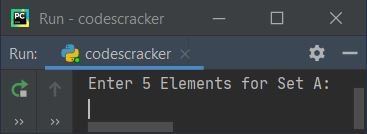Now supply any five elements for first set (Set A) say 1, 2, 3, 4, 5 and then five more elements for second set (Set B) say 6, 2, 7, 4, 8. Then program produces the output that shows the union of two given sets like shown in the snapshot given below:#### Modified Version of Previous Program

This is the modified version of previous program. This program allows user to define the size of both set along with its elements. The end= skips inserting an automatic newline.

```print("Enter Size of Set A: ", end="")
try:
tota = int(input())
print("Enter", tota, "Elements: ", end="")
numsOne = []
for i in range(tota):
numsOne.append(input())
setOne = set(numsOne)

print("\nEnter Size of Set B: ", end="")
try:
totb = int(input())
print("Enter", totb, "Elements: ", end="")
numsTwo = []
for i in range(totb):
numsTwo.append(input())
setTwo = set(numsTwo)

unionSet = setOne | setTwo
print("\nSet A: ", "{", ', '.join(setOne), "}")
print("Set B: ", "{", ', '.join(setTwo), "}")
print("\nUnion of A and B =", "{", ', '.join(unionSet), "}")
except ValueError:
print("\nInvalid Input!")
except ValueError:
print("\nInvalid Input!")```

Here is its sample run with user input 3 as size of first set (set A), then 1, 2, 3 as three elements, and 4 as size of second set (set B), then 1, 2, 3, 4 as its four elements: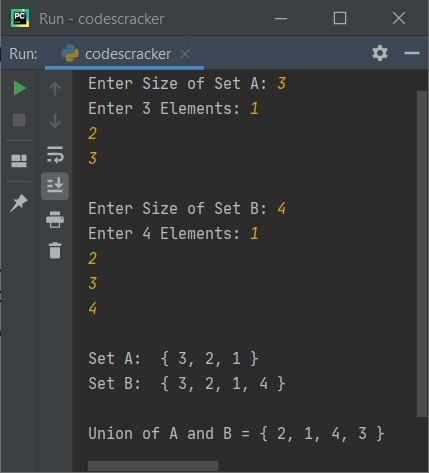Note - The join() in above program is used in a way, to remove ' from all elements of set.

## Find Intersection of Sets

Intersection of two sets (A and B) can be calculated as, all common elements from both the set.

To create a Python program that finds intersection of sets given by user at run-time just like the previous program that finds union. Follow the previous program with only two replacements. That is, first replace the following statement (from previous program):

`unionSet = setOne | setTwo`

with the statement given below:

`intersectionSet = setOne & setTwo`

And then replace the following (second) statement (from above program):

`print("\nUnion of A and B =", "{", ', '.join(unionSet), "}")`

with the statement given below:

`print("\nIntersection of A and B =", "{", ', '.join(intersectionSet), "}")`

Now here is its sample run with same user input as of previous program's sample run: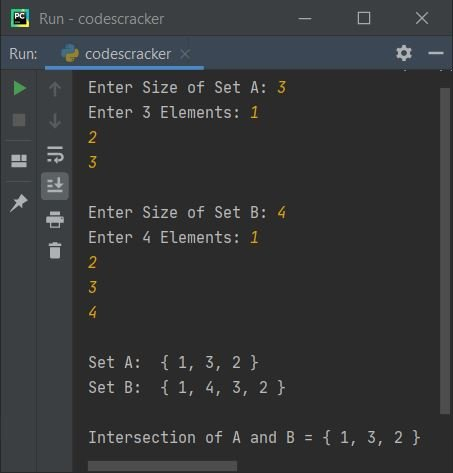## Find Difference of Sets

Difference of two sets (A and B) can be calculated as, all those elements that are present in set A but not in set B

To find difference of sets in Python, do the similar thing as done previously. That is, change the following two statements from the program given in modified version of union of set program. That is, replace the following statement:

`unionSet = setOne | setTwo`

with:

`diffSet = setOne - setTwo`

and then replace the second statement, that is:

`print("\nUnion of A and B =", "{", ', '.join(unionSet), "}")`

with:

`print("\nDifference of A and B =", "{", ', '.join(diffSet), "}")`

Here is its sample with user input, 5 as size of first set, then 1, 2, 3, 4, 5 as its five elements and 2 as size of second set, then 1, 6 as its two elements: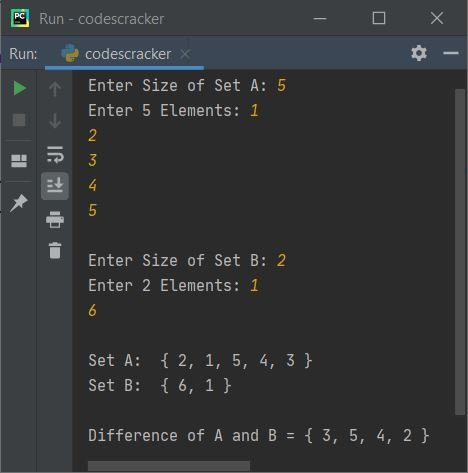## Find Symmetric Difference of Sets

Symmetric difference of two sets (A and B) can be calculated as, all unique elements from both the set.

Like done previously, in this case also, change the two statements with following two statements one by one:

```symDiffSet = setOne ^ setTwo
print("\nSymmetric Difference of A and B =", "{", ', '.join(symDiffSet), "}")```

Here is its sample run with user inputs 4 as size of set A, then 5, 6, 7, 8 as its four elements, and 3 as size of set B, then 1, 2, 7 as its three elements: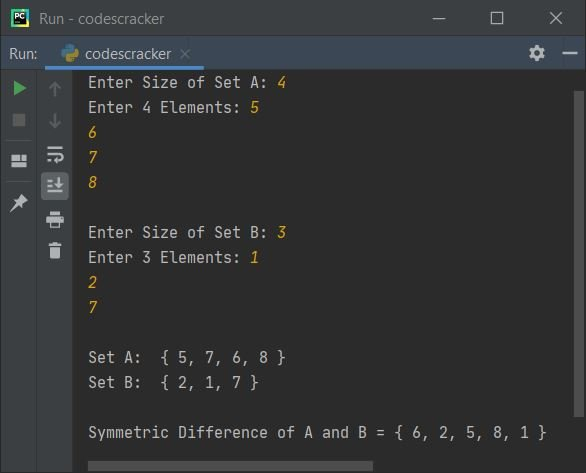## Find all Four Operations on Two Sets

This program is the combine version of previous four programs, that find and prints all the four operations of two given sets.

```print("Enter Size of Set A: ", end="")
try:
tota = int(input())
print("Enter", tota, "Elements: ", end="")
numsOne = []
for i in range(tota):
numsOne.append(input())
setOne = set(numsOne)

print("\nEnter Size of Set B: ", end="")
try:
totb = int(input())
print("Enter", totb, "Elements: ", end="")
numsTwo = []
for i in range(totb):
numsTwo.append(input())
setTwo = set(numsTwo)

unionSet = setOne | setTwo
intersectionSet = setOne amp; setTwo
diffSet = setOne - setTwo
symDiffSet = setOne ^ setTwo
print("\nSet A: ", "{", ', '.join(setOne), "}")
print("Set B: ", "{", ', '.join(setTwo), "}")
print("\nUnion of A and B =", "{", ', '.join(unionSet), "}")
print("\nIntersection of A and B =", "{", ', '.join(intersectionSet), "}")
print("\nDifference of A and B =", "{", ', '.join(diffSet), "}")
print("\nSymmetric Difference of A and B =", "{", ', '.join(symDiffSet), "}")
except ValueError:
print("\nInvalid Input!")
except ValueError:
print("\nInvalid Input!")```

Here is its sample run:Python Online Test

« Previous Program Next Program »